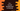# C++ program to convert hexadecimal to decimal## C++ program to convert hexadecimal to decimal:

In this post, we will learn how to convert a hexadecimal value to decimal. Hexadecimal and decimal both are different number system. The hexadecimal number system uses 16 as the base and decimal number system uses 10 as the base.

In hexadecimal number system, 0 to 9 and A to F are used for 10 to 15. If we need to convert a number from hexadecimal to decimal, we need to follow a simple algorithm. This algorithm can be used in any other programming language as well.

Let’s learn how this algorithm works with example.

### Algorithm to convert hexadecimal to decimal:

We can follow the following algorithm to convert a hexadecimal value to decimal:

• Take the hexadecimal value as an input from the user.
• Initialize a result variable as 0.
• Start from the rightmost digit to the first digit, the index of the rightmost digit is 0:
• Multiply the current digit value with 16 to the power of current index value. For example, for the rightmost digit, we will multiply it with 16^0. For 0 to 9, we will consider them as 0 to 9 and for A to F, we will consider these as 10 to 15.
• Add the multiplication value to the result variable.
• Once the iteration is done, the result will hold the final result.

### Example:

Let’s say we want to convert the hexadecimal value A4E8 to decimal.

• Start from the rightmost digit.
• Initialize result as 0.
• Multiply 8 with 16^0 = 8 * 16^0 = 8, So, result is 0 + 8
• Multiply E or 14 with 16^1 = 14 * 16^1 = 224, So, result is 8 + 224 = 232
• Multiply 4 with 16^2 = 4 * 16^2 = 1024, So, result is 232 + 1024 = 1256
• Multiply A or 10 with 16^3 = 10 * 16^3 = 40960, So, result is 1256 + 40960 = 42216

So, the decimal value of A4E8 is 42216.

### C++ program to convert a hexadecimal value to decimal:

Let’s write down a program to convert a hexadecimal value to decimal in C++:

``````#include <iostream>
#include <string>
using namespace std;

int convertHexToDecimal(char c)
{
if (c >= '0' && c <= '9')
{
return int(c) - 48;
}
else if (c >= 'A' && c <= 'F')
{
return int(c) - 55;
}
else if (c >= 'a' && c <= 'f')
{
return int(c) - 87;
}
else
{
return -1;
}
}

int main()
{
string hexValue = "a4e8";
int result = 0;
int multiplier = 1;

for (int i = hexValue.length() - 1; i >= 0; i--)
{
result += convertHexToDecimal(hexValue[i]) * multiplier;
multiplier *= 16;
}

cout << result << endl;
return 0;
}``````

Here,

• hexValue is the given string. It is the hexadecimal value we need to convert to decimal.
• result variable is initialized as 0 to hold the final result.
• multiplier is initialized as 1. It is the multiplier to multiply with the current digit of the number.
• The for loop iterates from the last character index to the first character index of the hexadecimal string.
• For each character, it calls convertHexToDecimal method to get the decimal value and multiplies it with the multiplier.
• It also updates the multiplier by multiplying it with 16 for the next iteration.
• The convertHexToDecimal method checks for both uppercase and lowercase characters. So, it will convert hexadecimal values with uppercase or lowercase characters.
• At the end of the program, it prints the decimal value calculated.

### Sample output:

If you run this program, it will give output as like below:

``42216``

If you want to calculate it for a different hexadecimal value, you need to update the value of hexValue. It will calculate the result for that hexadecimal string. It will give same result for both uppercase and lowercase hexadecimal values, e.g. it will print the same value for a4e8 and A4E8.